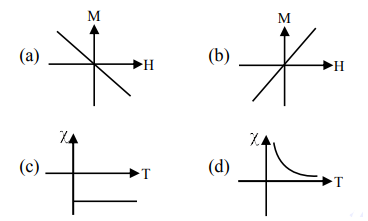# Following plots show Magnetization (M) vs Magnetising field (H) and Magnetic susceptibility ( F ) vs temperature (T) graph :`
Question:

Following plots show Magnetization (M) vs Magnetising field (H) and Magnetic susceptibility ( F ) vs temperature (T) graph :Which of the following combination will be represented by a diamagnetic material?

1. (a), (c)

2. $(a),(d)$

3. $(b),(d)$

4. $(b),(c)$

Correct Option: 1,

Solution:

Conceptual question Option (1)